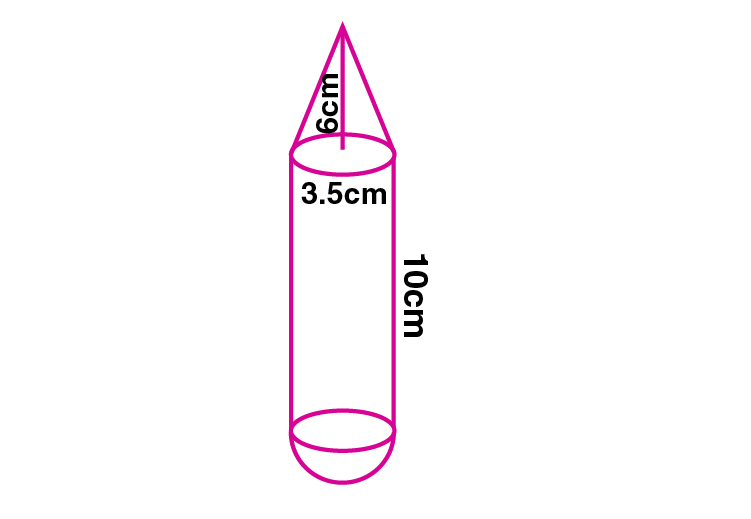Newbie

# A solid is in the form of a right circular cylinder with a hemisphere at one end and a cone at the other end. Their common diameter is 3.5 cm and the height of the cylindrical and conical portions are 10 cm and 6 cm respectively. Find the volume of the solid. (Take pi = 3.14)

• 0

An Important Question of class 10 Based on Mensuration Chapter of M.L Aggarwal for ICSE BOARD.
A solid is in the form of a right circular cylinder with a hemisphere at one end and a cone at the other end. Their common diameter and the height of the cylindrical and conical portions are given. Find the volume of the solid.
This is the Question Number 20, Exercise 17.4 of M.L Aggarwal.

Share

1.Given height of the cylinder, H = 10 cm

Height of the cone, h = 6 cm

Common diameter = 3.5 cm

Common radius, r = 3.5/2 = 1.75 cm

Volume of the solid = Volume of the cone + Volume of the cylinder + Volume of the hemisphere

= (1/3)r2h +r2H + (2/3) r3

= r2((h/3) + H + (2r/3))

= 3.14×1.752×((6/3)+10+(2×1.75)/3)

= 3.14×3.0625×(2+10+1.167)

= 3.14×3.0625×13.167

= 9.61625×13.167

= 126.617 cm3

= 126.62 cm3

Hence the volume of the solid is 126.62 cm3.

• 2